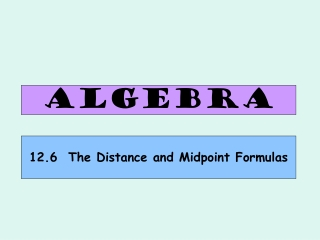DownloadDownload PresentationALGEBRA

# ALGEBRA

Télécharger la présentation## ALGEBRA

- - - - - - - - - - - - - - - - - - - - - - - - - - - E N D - - - - - - - - - - - - - - - - - - - - - - - - - - -
##### Presentation Transcript

1. ALGEBRA 12.6 The Distance and Midpoint Formulas

2. The Distance Formula The distance d between points and is: Why? Let’s try an example to find out! (-3, 4). Find the distance between (–3, 4) and (1, –4). 4 8 . (1, -4) 4√5 Pythagorean Theorem!

3. Examples Find the distance between the two points. Leave answers in simplified radical form. 1. A (3,5) B (7,8) Distance of AB = 5 √ √ d = + = (7 – 3)² (8 – 5)² 16 + 9 =√25 = 5 2. C (-7,2) D (-2,-10) Distance of CD = 13 √ + = √ d = (-10 – 2)² 25 + 144 (-2 +7)² =√169 = 13

4. Example Decide whether the points (6,4), (-3,1) and (9,-5) are vertices of a right triangle. √ √ = d1 = + (6 + 3)² (4 – 1)² 81 + 9 =√90 = 3√10 √ √ = d2 = + = √180 = 6√5 (-3 - 9)² (1 + 5)² 144 + 36 √ √ = d3 = + = √90 = 3√10 (6 - 9)² (4 + 5)² 9 + 81 Now use the Pythagorean Theorem Converse to check. Does the sum of the squares of the two shorter sides equal the square of the longest side? short² short² long² (3√10)² + (3√10)² = (6√5)² 90 + 90 = 180 Yes. It is a right triangle. 180 = 180

5. The Midpoint Formula The midpoint of the segment that joins points (x1,y1) and (x2,y2) is the point • (6,8) • (1,5) • (-4,2)

6. How does it work? Find the coordinate of the Midpoint of BC. B (12,7) C (4,1) A B Midpoint: 7 ● ● 12 + 4 7 + 1 4 ● , 2 2 1 C ● 4 8 12 (8,4)

7. Exercises 1. A (3,5) B (7,-5) 3+7 5+(-5) (5,0) midpoint: , 2 2 2. A (0,4) B (4,3) 0+4 4+3 midpoint: 7 , (2, ) 2 2 2

8. Exercise There are 90 feet between consecutive bases on a baseball diamond. Suppose 3rd base is located at (10,0) and first base is located at (100,-90). A ball is hit and lands halfway between first base and third base. Where does the ball land? Sketch it. 2nd ● 3rd 1st (10,0) (100,-90) 10 + 100 0 - 90 midpoint: , home 2 2 (55,-45)

9. Homework pg. 748 #15-45 odd #54,55